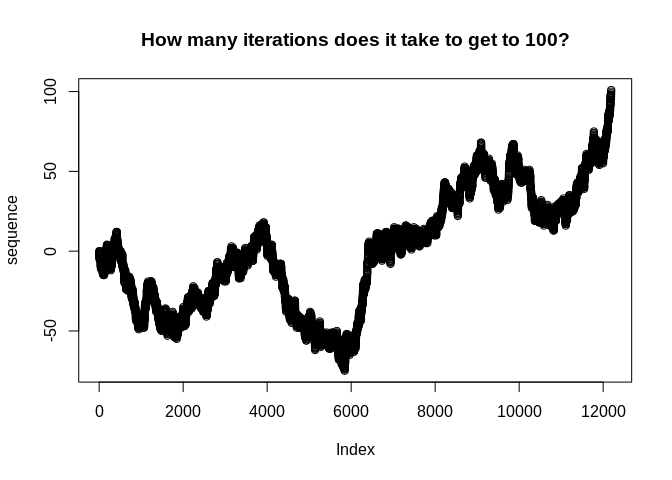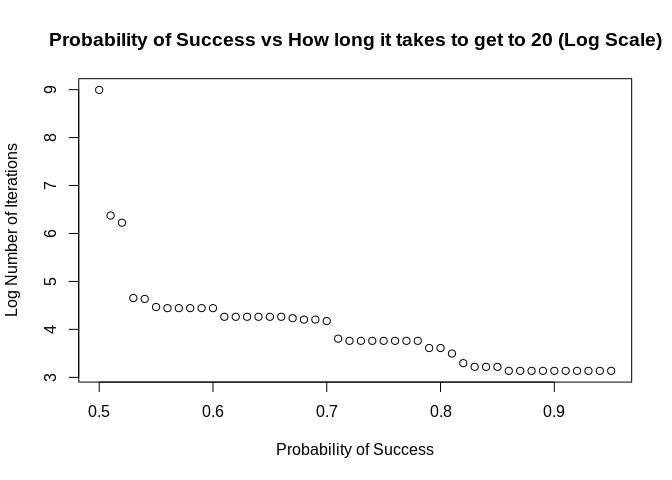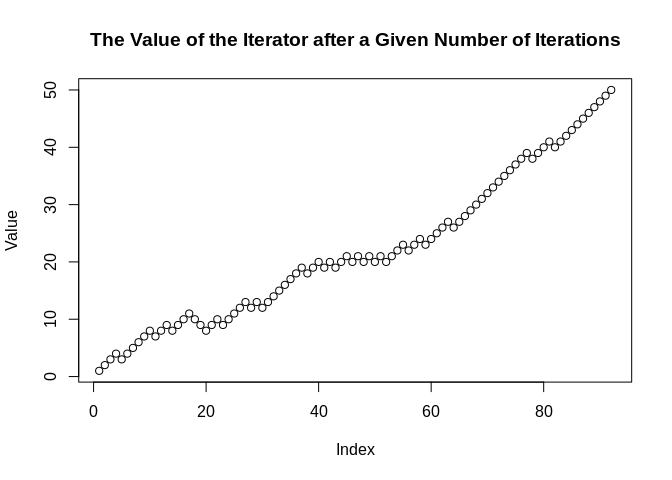# peruse

The {peruse} package is aimed at making it easier to generate irregular sequences that are difficult to generate with existing tools.

The heart of {peruse} is the `S3` class `Iterator`. An `Iterator` allows the user to write an arbitrary R expression that returns the next element of a sequence of R objects. It then saves the state of the `Iterator`, meaning the next time evaluation is invoked, the initial state will be the result of the previous iteration. This is most useful for generating recursive sequences, those where each iteration depends on previous ones.

The package also provides a simple, tidy API for set building, allowing the user to generate a set consisting of the elements of a vector that meet specific criteria. This can either return a vector consisting of all the chosen elements or it can return an `Iterator` that lazily generates the chosen elements.

At the end of this document, there is a tutorial for metaprogramming (that is, programmatically generating code) with `Iterator`s.

## Installation

You can install the released version of peruse from CRAN with:

``install.packages("peruse")``

And the development version from GitHub with:

``````# install.packages("devtools")
devtools::install_github("jacgoldsm/peruse")``````

## Example

### Negative Binomial Investigation

Suppose we want to investigate the question of how many trials it takes for a random walk with drift to reach a given threshold. We know that this would follow a Negative Binomial distribution, but how could we use the Iterator to look at this empirically in a way that easily allows us to adjust the drift term and see how the result changes? We might do something like this:

``````p_success <- 0.5
threshold <- 100

iter <- Iterator({
set.seed(seeds[.iter])
n <- n + sample(c(1,-1), 1, prob = c(p_success, 1 - p_success))
},
list(n = 0, seeds = 1000:1e5),
n)
sequence <- yield_while(iter, n <= threshold)

plot(sequence, main = "How many iterations does it take to get to 100?")``````How would we apply this same function to a grid of probabilities? We could do something like this:

``````probs <- seq(0.5,0.95, by = 0.01)
num_iter <- rep(NA, length(probs))
threshold <- 20
seeds <- 1000:1e6

for (i in seq_along(probs)) {
iter <- Iterator({
set.seed(seeds[.iter])
n <- n + sample(c(1,-1), 1, prob = c(!! probs[i], 1 - !! probs[i]))
},
list(n = 0),
yield = n)

num_iter[i] <- length(yield_while(iter, n <= threshold))
}

plot(x = probs,
y = log(num_iter),
main = "Probability of Success vs How long it takes to get to 20 (Log Scale)",
xlab = "Probability of Success",
ylab = "Log Number of Iterations")``````Alternatively, using functional programming:

``````make <- function(p) {
iter <- Iterator({
set.seed(seeds[.iter])
n <- n + sample(c(1,-1), 1, prob = c(!! p, 1 - !! p))
},
list(n = 0),
yield = n)

length(yield_while(iter, n <= threshold))
}

num_iter <- sapply(seq(0.5,0.95, by = 0.01), make)``````

This illustrates a few useful features of Iterators:

• We can use environment variables in either our expression or our `while` condition to represent constants. In this case, `threshold` doesn’t change between iterations or between parameters. If you are creating many `Iterator`s, it can be faster to use environment variables, since you don’t have to make a new object for each new `Iterator`.

• We can use the forcing operators from `{rlang}` (`!!`) to force evaluation of arguments in place, in this case substituting the expression of `probs[i]` with the value of `probs[i]` (see the end of this document for a tutorial on metaprogramming with `Iterator`s).

• We can refer to the current iteration number in `yield_while()`, `yield_more()`, or their silent variants with the variable `.iter`.

### Collatz Sequence

A Collatz sequence is a particular sequence of natural numbers that mathematicians think always reaches 1 at some point, no matter the starting point. We can’t prove that one way or the other, but we can create an `Iterator` that lazily generates a Collatz sequence until it reaches 1:

``````library(peruse)

# Collatz generator starting at 50
collatz <- Iterator({
if (n %% 2 == 0) n <- n / 2 else n <- n*3 + 1
},
initial = list(n = 50),
yield = n)

yield_while(collatz, n != 1L)
#>   25 76 38 19 58 29 88 44 22 11 34 17 52 26 13 40 20 10  5 16  8  4  2  1``````

### Random Walk with Drift

Random Walks, with or without drift, are one of the most commonly used type of stochastic processes. How can we simulate one with {peruse}?

``````rwd <- Iterator({
n <- n + sample(c(-1L, 1L), size = 1L, prob = c(0.25, 0.75))
},
initial = list(n = 0),
yield = n)

Value <- yield_while(rwd, n != 50L & n != -50L)

plot(Value, main = "The Value of the Iterator after a Given Number of Iterations")``````Here, we can see that `seq` gets to `50` after about `100` iterations when it is weighted `3:1` odds in favor of adding `1` over adding `-1` to the prior value.

## A Note

Helper functions `yield_more()`, `yield_while()`, `move_more()`, and `move_while()` behave mostly like syntactic sugar for explicit loops. So,

``````it <- Iterator(x <- x + 1L, list(x = 0), x)
it2 <- clone(it)
x <- numeric(100)
for (i in 1:100) {
x[i] <- yield_next(it)
}``````

will give the same result as

``y <- yield_more(it2, 100)``

However, doing it the latter way is significantly more efficient than the former. This is because a lot of the overhead only needs to be done once per call to `yield`. That means that a lot less has to be done on every iteration when you explicitly call `yield_more()`.

This is even more true with `yield_while()`. Pretend we don’t know how long this `Iterator` will take to reach 10,000. Doing something like:

``````x <- numeric()
while (it\$initial\$x < 10000) {
x <- c(x, yield_next(it))
}``````

is very inefficient because it requires reallocating the vector on every iteration. On the other hand, the following is both easier to read and much more efficient:

``y <- yield_while(it2, x < 10000)``

Internally, `yield_while()` uses an efficient algorithm for resizing the result in linear amortized time. As a result, it will evaluate much faster.

### Primes

How about generating all the prime numbers between `1` and `100`? We can easily do that with the set-builder API:

``````2:100 %>%
that_for_all(range(2, .x)) %>%
we_have(~.x %% .y)
#>    2  3  5  7 11 13 17 19 23 29 31 37 41 43 47 53 59 61 67 71 73 79 83 89 97``````

In the equation, we can reference the left-hand side of the equation with the positional variable `.x`, and the right-hand side (that is, the argument in `that_for_all()`) with `.y`. The equation can be anything recognized as a function by `rlang::as_function()`.

But how about if we want to generate the first 100 prime numbers? We don’t know the range of values this should fall in (well, mathematicians do), so we can use laziness to our advantage:

``````primes <- 2:10000 %>%
that_for_all(range(2, .x)) %>%
we_have(~.x %% .y, "Iterator")

primes_2 <- clone(primes)``````

The first prime number is

``````yield_next(primes_2)
#>  2``````

And the first 100 are:

``````sequence <- yield_more(primes, 100)
sequence
#>      2   3   5   7  11  13  17  19  23  29  31  37  41  43  47  53  59  61
#>    67  71  73  79  83  89  97 101 103 107 109 113 127 131 137 139 149 151
#>   157 163 167 173 179 181 191 193 197 199 211 223 227 229 233 239 241 251
#>   257 263 269 271 277 281 283 293 307 311 313 317 331 337 347 349 353 359
#>   367 373 379 383 389 397 401 409 419 421 431 433 439 443 449 457 461 463
#>   467 479 487 491 499 503 509 521 523 541``````

Here, we use `clone()` to create an identical `Iterator` to `primes` that can be modified separately.

`clone()` also carries optional arguments that override the `\$initial` parameters in the old `Iterator`. For example,

``````it <- Iterator({m <- m + n}, list(m = 0, n = 1), m)
it2 <- clone(it, n = 5)
yield_next(it)
#>  1
yield_next(it2)
#>  5``````

Here, we overrode `n = 1` in `it` with `n = 5` in `it2`.

## Set Utilities

`Iterator`s that are created from set comprehension have several utilities:

• Refer to the vector `.x` with the variable `.x_vector`

• Refer to the current index of `.x_vector` with `.i` (not to be confused with `.iter`).

Here is an example of putting those together to yield to the end of the sequence:

``````primes_100 <- 2:100 %>%
that_for_all(range(2, .x)) %>%
we_have(~.x %% .y, "Iterator")

yield_while(primes_100, .x_vector[.i] <= 100)
#> (Note: result has reached end of sequence)
#>    2  3  5  7 11 13 17 19 23 29 31 37 41 43 47 53 59 61 67 71 73 79 83 89 97``````

As you can see, the sequence terminates with a message that the end has been reached.

In reality, the sequence will terminate at the end anyway, so you can generate the whole sequence like this:

``````primes_100 <- 2:100 %>%
that_for_all(range(2, .x)) %>%
we_have(~.x %% .y, "Iterator")

yield_while(primes_100, T)
#> (Note: result has reached end of sequence)
#>    2  3  5  7 11 13 17 19 23 29 31 37 41 43 47 53 59 61 67 71 73 79 83 89 97``````

### Missing Values

Set comprehension does not specially handle missing values. All the elements of set `.x` will be compared with all the elements of set `.y` by `formula`, and the value of `.x` will be included if and only if the condition returns `TRUE`. If the comparison returns `NA`, the expression will terminate with an error.

Be aware of two things: First, expressions like `NA == NA`, `NA > NA`, and `NA <= NA` return `NA`. Second, expressions of the form `if (NA) action` are illegal and will result in an error.

As a result, an expression like this will not work:

``c(2:20, NA_integer_) %>% that_for_all(range(2, .x)) %>% we_have(~ .x %% .y)``

In fact, this will fail for two reasons: `range(2, .x)` will not work when `.x` is `NA`, and the comparison `if (NA %% 2)` will also not work.

Normally, you will want to drop `NA` values from your vectors before using set comprehension. If you are careful, you can write valid code with `NA`s, but it will be very painful by comparison:

``````c(2:20, NA_integer_) %>%
that_for_all(if (is.na(.x)) NA else range(2, .x)) %>%
we_have(~ is.na(.x) || .x %% .y)
#>   2  3  5  7 11 13 17 19 NA``````

Here, we avoid `range(2, NA)` with our conditional, and avoid having `NA` in the `if` statement in `we_have()` by making sure to return `TRUE` when `.x` is missing.

# Tutorial: Metaprogramming with `Iterator`s

`Iterator`s are designed to be flexible, almost as flexible as ordinary R functions. They are also designed to be tidy, using tools from the “Tidyverse” family of R extensions. Unfortunately, those goals are not entirely compatible when it comes to metaprogramming, leading to a sort of “semi-tidy” evaluation. Use these examples as a reference for programmatically generating `Iterator` expressions.

In almost all cases, the environment in which an Iterator is made does not effect its execution; rather, the environment from which `yield_next()` or its cousins is called determines evaluation. In this way, it is similar to ordinary R functions. The one small exception will be detailed at the end.

### Forcing

Use `!!` to force evaluation of names, just like you would in `dplyr` or any Tidyverse function:

``````p <- 0.5
i <- Iterator({x <- x + !! p}, list(x = 0), x)
yield_more(i, 5)
#>  0.5 1.0 1.5 2.0 2.5``````

There is no built-in mechanism to force evaluation of names in the `\$initial` list, but you can use tools like `rlang::list2()` to do so. `\$initial` can be anything coercible to list.

``````p <- 0.1
x <- as.symbol("my_var")
i <- Iterator({!! x <- !! x + !! p}, rlang::list2(!! x := 0), !! x)
yield_more(i, 5)
#>  0.1 0.2 0.3 0.4 0.5``````

Note the use of the “walrus” operator (`:=`) to assign names in `rlang::list2()`, see the documentation in `rlang::nse-force()` for more details.

### Force-defuse

Function arguments are a special data structure in R. They really represent up to three different things: the name given to the argument in the function, possibly the name of the argument when the function is called if it is named, and the value of the argument passed to the function. Since `Iterator`s don’t use quosures (because they work independently of the environment where they are created), you can’t use `{{ }}` to force-defuse expressions.

• To get a variable name from a parameter name, `substitute()` the variable at the beginning.

• If you want the value of the variable, just leave it be.

Then use the `bang-bang` (!!) operator to add all of them to the `Iterator`:

``````make_random_walk_with_drift <- function(drift, variable) {
variable <- substitute(variable)  # creates a variable whose value is x
Iterator({
!! variable <- !! variable + sample(c(-1,1), 1, TRUE, c(!! drift, 1 - !! drift))
},
initial = rlang::list2(!! variable := 0), !! variable)
}

yield_more(make_random_walk_with_drift(0.5, x), 5)
#>   1  2  1  0 -1``````

### Indirection

Since `Iterator`s don’t use data masks, they don’t have `.data` and `.env` pronouns. If you have a variable in `iter\$initial` and a variable with the same name in your global environment, just force immediate evaluation of the environment variable with `!!`.

### One Exception

Ordinarily, `Iterator`s work independently from the environment in which they were created. The one exception is when an `Iterator` is created from the template,

``iterator <- .x %>% expression_with_.x %>% we_have(formula, "Iterator")``

the variable `expression_with_.x` is turned into a quosure. That means that it will always be evaluated in the environment where `iterator` was made.

For a concrete example, consider:

``````offset <- 2
it <- 2:100 %>% that_for_all(range(offset, .x)) %>% we_have(~ .x %% .y, "Iterator")
fun <- function() {
offset <- 3
yield_while(it, !.finished) # print the whole sequence
}

fun()
#> (Note: result has reached end of sequence)
#>    2  3  5  7 11 13 17 19 23 29 31 37 41 43 47 53 59 61 67 71 73 79 83 89 97``````

We can see that the code does not select elements that are divisible by 2 but not any other numbers, as would be the case with offset equal to three. Our expression `range(offset, .x)` is evaluated in the global environment, not in the execution environment of `fun()`.

# Citation

This software contains a modified version of a small piece of code from the `purrr` package, by Hadley Wickham, Lionel Henry, and RStudio, freely available under the MIT License.